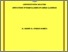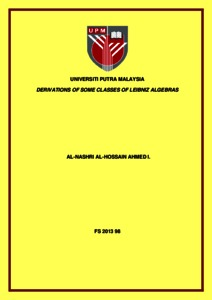# Derivations of some classes of Leibniz algebras

## Citation

Ahmed I, Al-Nashri Al-Hossain (2013) Derivations of some classes of Leibniz algebras. Doctoral thesis, Universiti Putra Malaysia.

## Abstract

Let L be an algebra over a field K. It is called a Leibniz algebra if its the bilinear binary operation [ ; ] satisfies the following Leibniz identity: (x, [y, z]) = ([x, y]), z – ([x, z]), y , ∀ x, y, z ∈ L. The thesis deals with derivations of Liebniz algebras introduced by Loday in 1993. Leibniz algebra is a generalization of Lie algebra. The class of Leibniz algebras in dimensional two and three has been classified by Loday and Omirov, respectively. Starting from dimensions four and above there are classifications of subclasses (in dimension four nilpotent case and in dimensions (5-8) there are classifications of filiform Leibniz algebras). According to the classification results above, in the thesis the derivations of each of the classes are given. It is known that the class of filiform Leibniz algebras in dimension n is split into three disjoint sub classes denoted by F Lbn, SLbn and T Lbn. The main results of the research are follows: • An algorithm to find the derivations of any class of algebras is given, which is implemented to low-dimensional cases of Leibniz algebras. • Descriptions of derivations of low-dimensional Leibniz algebras (in dimensions two and three this description is given for all Leibniz algebras, in dimension four the derivations of nilpotent part are given and in dimensions 5 -8 the derivations of filiform Leibniz algebras are found). In each of the cases the Leibniz algebras which are characteristically nilpotent are identified. • The derivations of naturally graded Leibniz algebras is described and the derivation algebra dimensions are provided. • For the classes FLbn, SLbn and T Lbn estimations of dimensions of the derivation algebras, depending on n, are given . • A computer program for finding the derivations of algebras is provided.Preview
Text
FS 2013 98 IR.pdfView Item